CBSE Class 10 Sample Paper for 2023 Boards - Maths Standard

Class 10
Solutions of Sample Papers for Class 10 Boards

## (d) Assertion (A) is false but reason(R) is true.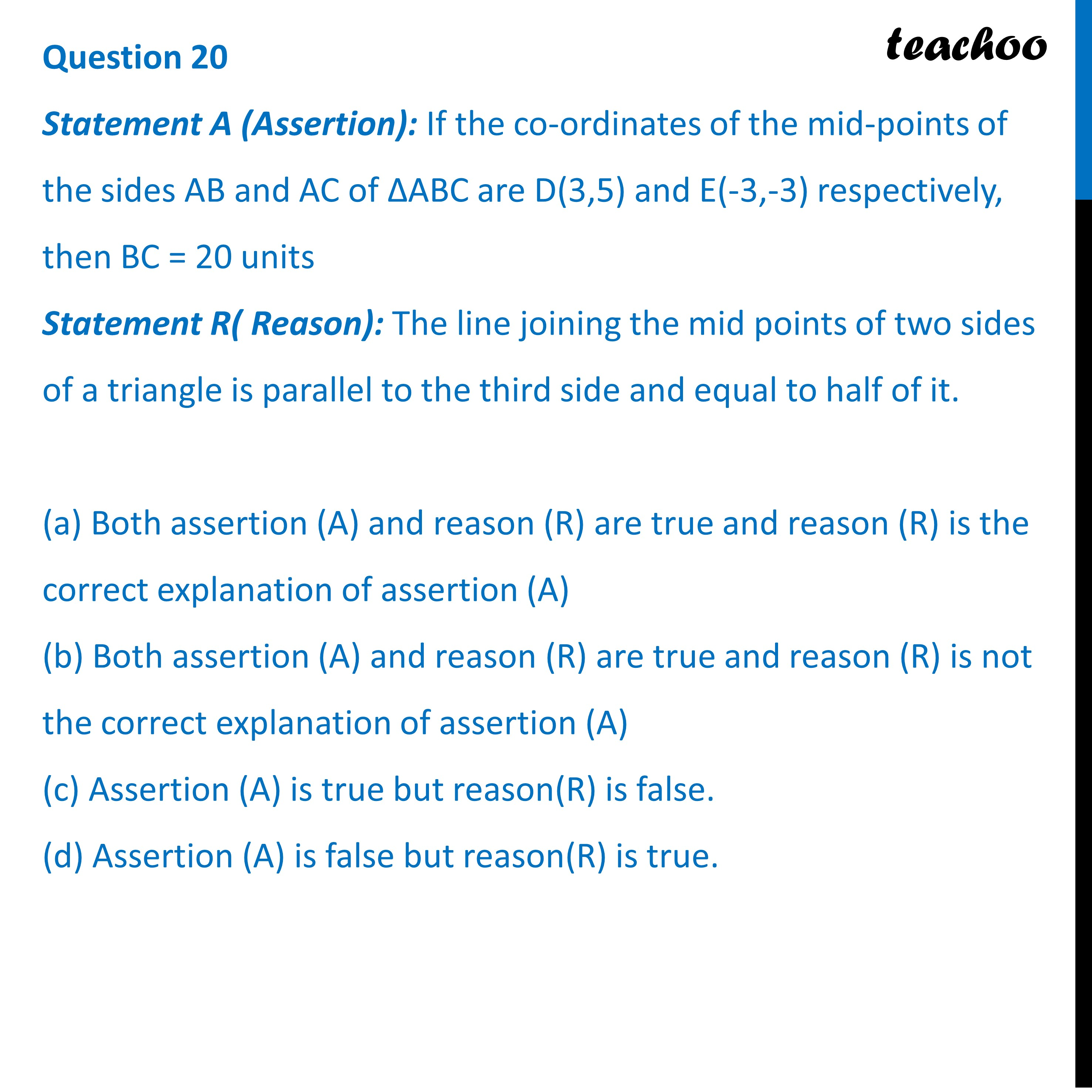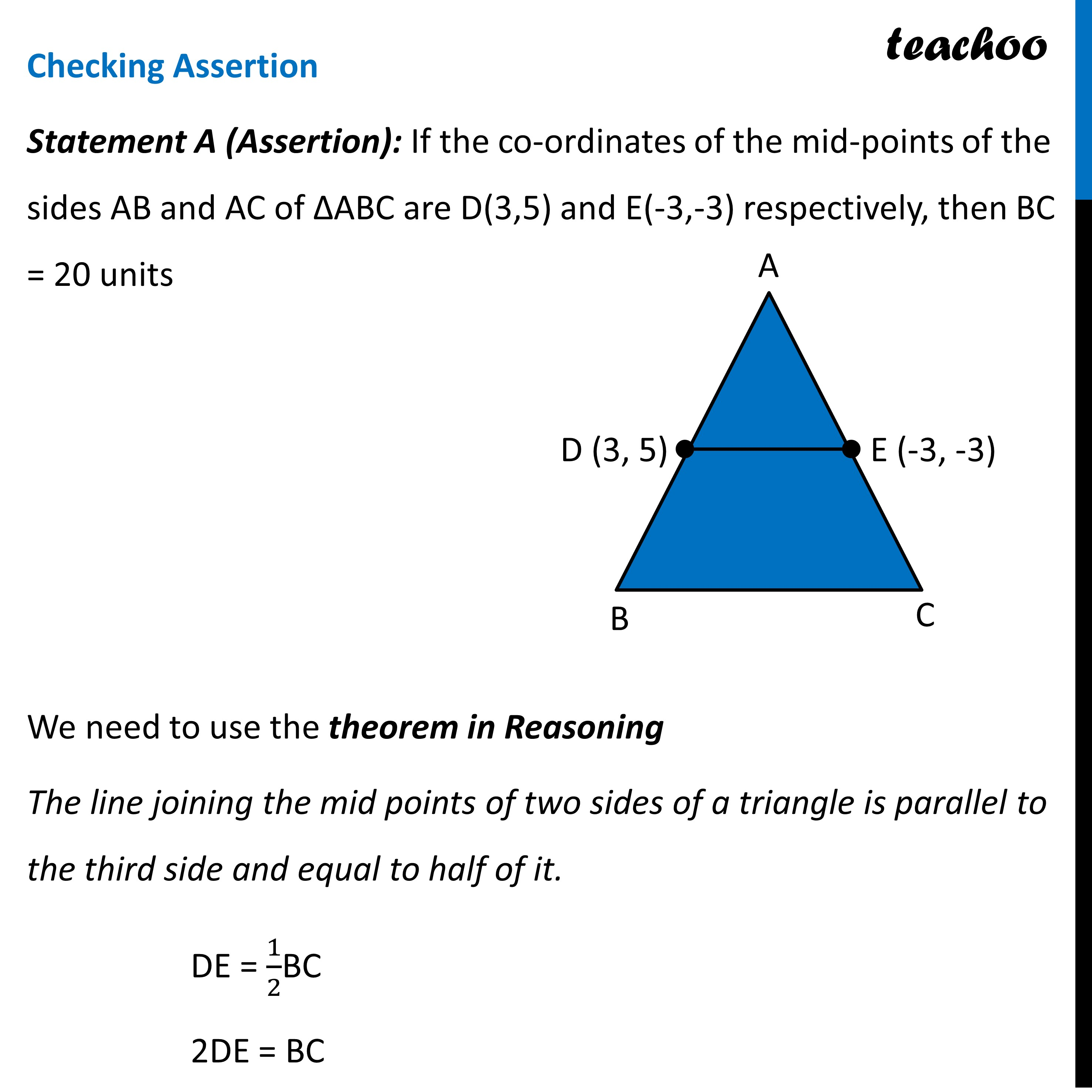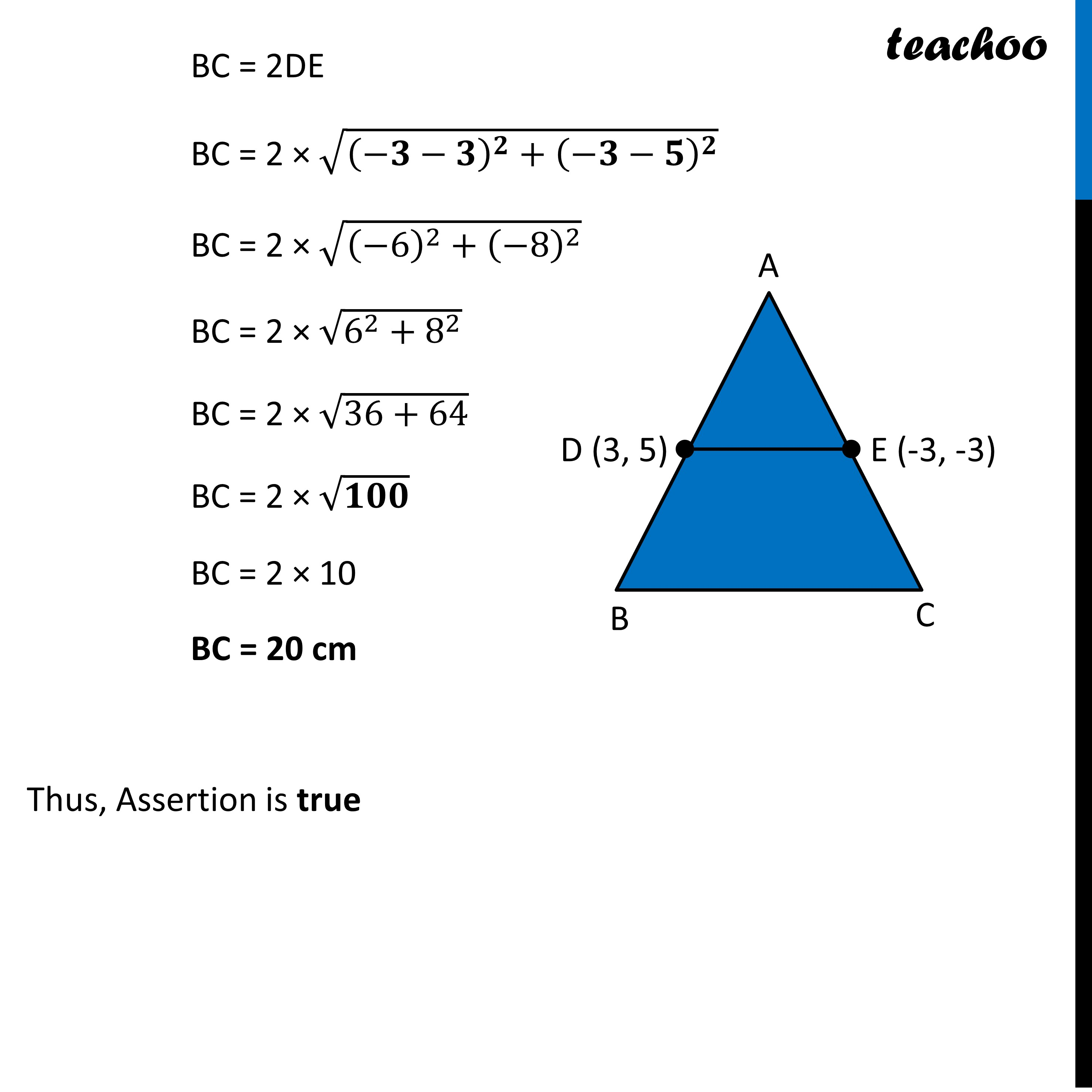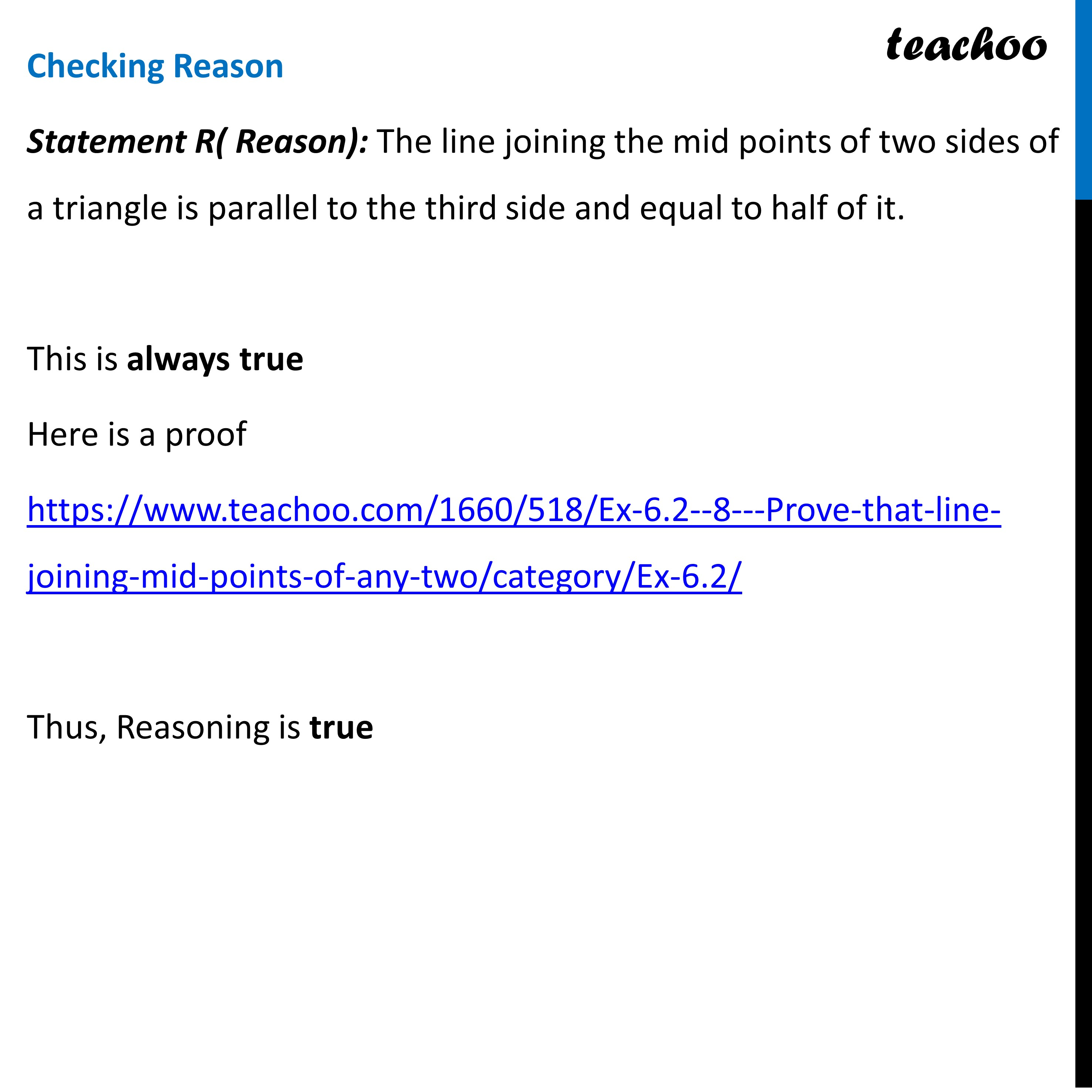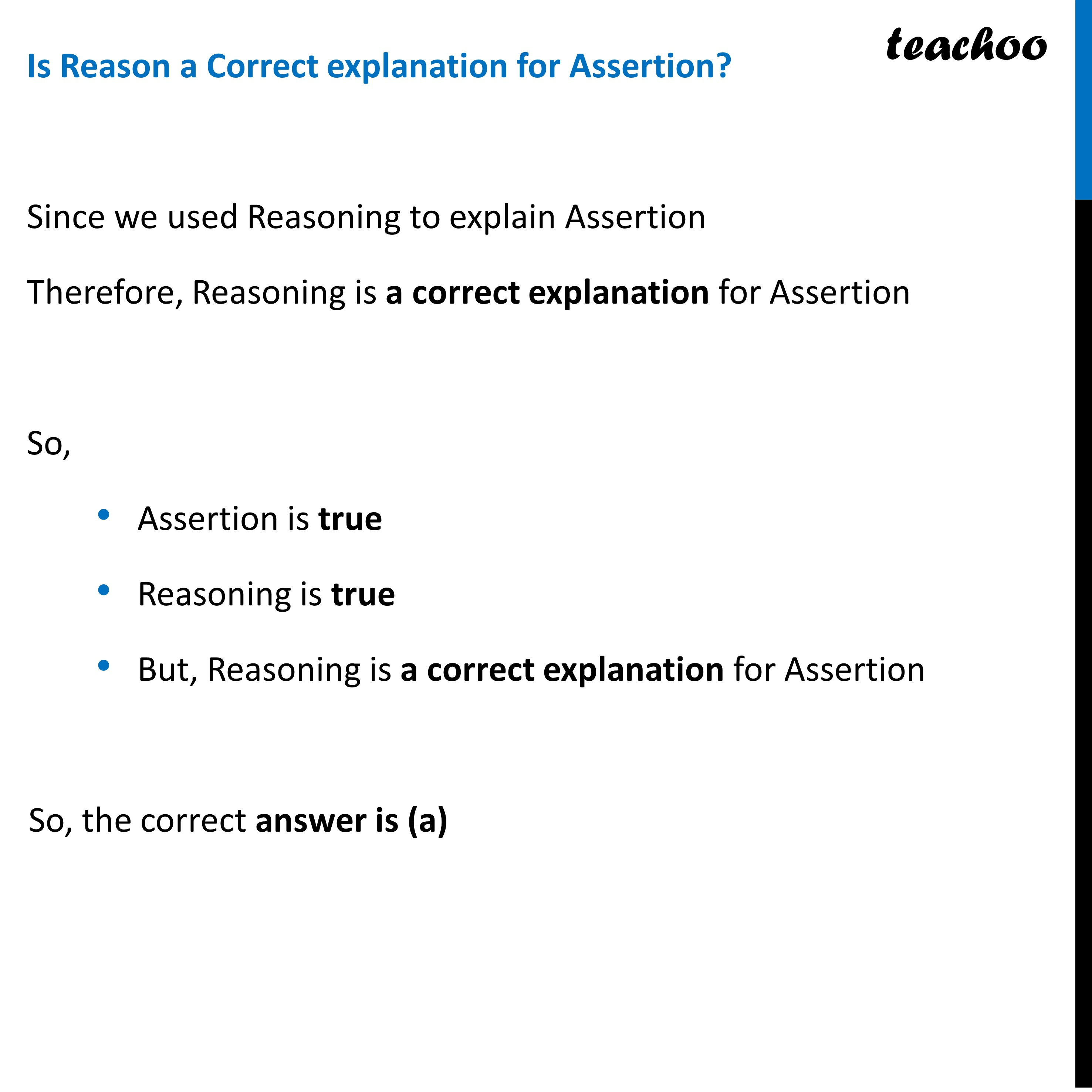Here is a proof

Learn in your speed, with individual attention - Teachoo Maths 1-on-1 Class

### Transcript

Question 20 Statement A (Assertion): If the co-ordinates of the mid-points of the sides AB and AC of ∆ABC are D(3,5) and E(-3,-3) respectively, then BC = 20 units Statement R( Reason): The line joining the mid points of two sides of a triangle is parallel to the third side and equal to half of it. (a) Both assertion (A) and reason (R) are true and reason (R) is the correct explanation of assertion (A) (b) Both assertion (A) and reason (R) are true and reason (R) is not the correct explanation of assertion (A) (c) Assertion (A) is true but reason(R) is false. (d) Assertion (A) is false but reason(R) is true.Checking Assertion Statement A (Assertion): If the co-ordinates of the mid-points of the sides AB and AC of ∆ABC are D(3,5) and E(-3,-3) respectively, then BC = 20 units We need to use the theorem in Reasoning The line joining the mid points of two sides of a triangle is parallel to the third side and equal to half of it. DE = 1/2BC 2DE = BC BC = 2DE BC = 2 × √((−𝟑−𝟑)^𝟐+(−𝟑−𝟓)^𝟐 ) BC = 2 × √((−6)^2+(−8)^2 ) BC = 2 × √(6^2+8^2 ) BC = 2 × √(36+64) BC = 2 × √𝟏𝟎𝟎 BC = 2 × 10 BC = 20 cm Thus, Assertion is true Checking Reason Statement R( Reason): The line joining the mid points of two sides of a triangle is parallel to the third side and equal to half of it. This is always true Here is a proof https://www.teachoo.com/1660/518/Ex-6.2--8---Prove-that-line-joining-mid-points-of-any-two/category/Ex-6.2/ Thus, Reasoning is true Is Reason a Correct explanation for Assertion? Since we used Reasoning to explain Assertion Therefore, Reasoning is a correct explanation for Assertion So, Assertion is true Reasoning is true But, Reasoning is a correct explanation for Assertion So, the correct answer is (a)